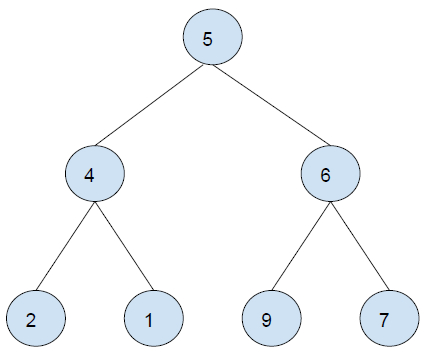# Find sum of all right leaves in a given Binary Tree in C++

C++Server Side ProgrammingProgramming

In this problem, we are given a binary tree. Our task is to find the sum of all left right in a given Binary Tree.

Let's take an example to understand the problem,

Input :Output : 8

Explanation

All leaf nodes of the tree are : 1, 8
Sum = 1 + 8 = 9

## Solution Approach

A simple solution to the problem is traversing the tree from root to leaf. If a node is a left leaf node, add it to sum. When the whole tree is traversed. Print Sum.

## Example

Program to illustrate the working of our solution

#include <iostream>
using namespace std;
struct Node{
int key;
struct Node* left, *right;
};
Node *newNode(char k){
Node *node = new Node;
node->key = k;
node->right = node->left = NULL;
return node;
}
bool isLeafNode(Node *node){
if (node == NULL)
return false;
if (node->left == NULL && node->right == NULL)
return true;
return false;
}
int findRightLeavesSum(Node *root){
int sum = 0;
if (root != NULL){
if (isLeafNode(root->right))
sum += root->right->key;
else
sum += findRightLeavesSum(root->right);
sum += findRightLeavesSum(root->left);
}
return sum;
}
int main(){
struct Node *root = newNode(5);
root->left = newNode(4);
root->right = newNode(6);
root->left->left = newNode(2);
root->left->right = newNode(1);
root->right->left = newNode(9);
root->right->right = newNode(7);
cout<<"The sum of right leaves of the tree is "<<findRightLeavesSum(root);
return 0;
}

## output

The sum of right leaves of the tree is 8

Another approach using Iteration

We will perform depth first search traversal on the tree, And then check if the current node is a right leaf. If Yes, add its value to sum, Otherwise, leave. At the end, print the sum.

## Example

Program to illustrate the working of our solution

#include<bits/stdc++.h>
using namespace std;
struct Node{
int key; struct Node* left, *right;
};
Node *newNode(char k){
Node *node = new Node;
node->key = k;
node->right = node->left = NULL;
return node;
}
int findRightLeavesSum(Node* root){
if(root == NULL) return 0;
stack<Node*> treeNodes;
treeNodes.push(root); int sum = 0;
while(treeNodes.size() > 0){
Node* currentNode = treeNodes.top();
treeNodes.pop();
if (currentNode->right != NULL){
treeNodes.push(currentNode->right);
if(currentNode->right->right == NULL &&
currentNode->right->left == NULL){
sum += currentNode->right->key ;
}
}
if (currentNode->left != NULL)
treeNodes.push(currentNode->left);
}
return sum;
}
int main(){
Node *root = newNode(5);
root->left= newNode(4);
root->right = newNode(6);
root->left->left = newNode(2);
root->left->right = newNode(1);
root->right->left = newNode(9);
root->right->right= newNode(7);
cout<<"The sum of right leaves of the tree is "<<findRightLeavesSum(root);
return 0;
}

## Output

The sum of right leaves of the tree is 8

Approach 3, using BFS

We will perform a breadth first search with a variable to indicate whether the node is the right leaf child or not. If it is, add it to the sum, else leave. At the end, print sum.

## Example

Program to illustrate the working of our solution

#include<bits/stdc++.h>
using namespace std;
struct Node{
int key; struct Node* left, *right;
};
Node *newNode(char k){
Node *node = new Node;
node->key = k;
node->right = node->left = NULL;
return node;
}
int findRightLeavesSum(Node* root) {
if (root == NULL)
return 0;
queue<pair<Node*, bool> > leftTreeNodes;
leftTreeNodes.push({ root, 0 });
int sum = 0;
while (!leftTreeNodes.empty()) {
Node* temp = leftTreeNodes.front().first;
bool is_left_child = leftTreeNodes.front().second;
leftTreeNodes.pop();
if (!temp->left && !temp->right && is_left_child)
sum = sum + temp->key;
if (temp->left) {
leftTreeNodes.push({ temp->left, 0 });
}
if (temp->right) {
leftTreeNodes.push({ temp->right, 1 });
}
}
return sum;
}
int main(){
Node *root = newNode(5);
root->left= newNode(4);
root->right = newNode(6);
root->left->left = newNode(2);
root->left->right = newNode(1);
root->right->left = newNode(9);
root->right->right= newNode(7);
cout<<"The sum of right leaves of the tree is "<<findRightLeavesSum(root);
return 0;
}

## Output

The sum of right leaves of the tree is 8